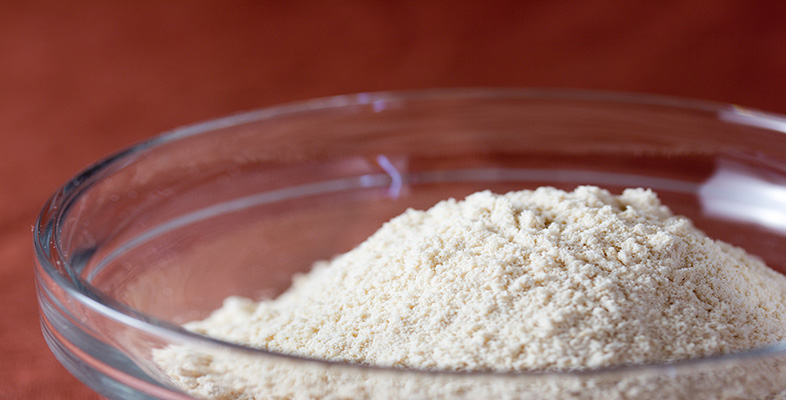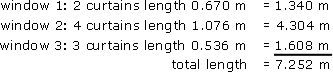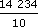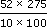Rounding and estimation

Start this free course now. Just create an account and sign in. Enrol and complete the course for a free statement of participation or digital badge if available.

Free course

# 3.5.1 Try some yourself

## Activity 27

Give the appropriate rounding for each of the values below:

• (a) Carpet floor area = 26.456 sq metres

• (b) Interest earned = £109.876 5439

• (c) Bill for £84.90 shared by 7 people

• (d) Length of room = 5.387 6956 m

• (e) Estimated attendance = 24.678

• (f) Thickness of paper = 0.0543 mm

• (a) 27 sq metres (Round up if you are buying carpet)

• (b) £109.88 (Money is given to 2 d.p.)

• (c) £12.13 (Round up to have enough money)

• (d) 5.39 m (Round to the nearest cm – depends on context)

• (e) 25 (Cannot have part of a person)

• (f) 0.05 mm (Cannot visualise more accuracy)

## Activity 28

A coach costs £85 to hire for a journey. There are six of you to share the cost. How much should you each pay?

85 ÷ 6 = 14.166 6667

You need to round up to cover the cost of the coach, and you need to round to the nearest penny at least. So £85 ÷ 6£14.17 or even £15 (including a tip for the driver).

## Activity 29

Suppose somebody baked two dozen fruit cakes for a charity sale and you want to charge a reasonable price for them. The cost of the ingredients, £40.86, is to be refunded to the cook. The organiser has suggested selling at a profit of about double this. What is a sensible selling price?

40.86 × 3 ÷ 24 = 5.1075.

You will probably want to charge a price that can easily be handled by buyers and sellers at the sale, and so rounding to the nearest pound or ten pence would be sensible, giving £5.00 or £5.10. However, a reduction to £4.99 might attract more buyers.

## Activity 30

Suppose that a friend has asked you to check the following calculations he has made for material for new curtains. Is anything wrong?

Cost at £3 per metre (length) is £10.956, i.e. approximately £11.

Your friend would have been better converting all the lengths to metres first, as there is an error in the total. You might have spotted this since 7.04 m plus two other lengths must give more than 3.652 m. There is also another error: 1 m 76 mm is 1.076 m; and 4 times this is 4.304 m.

An estimate of the total lengths would have suggested something was wrong. Nine curtains at between half a metre and a metre in length will need between 4and 9 metres. At £3 per metre this is well over £11.

Your friend also needs to round up the lengths of material to allow for hemming.

So the corrected calculation would be like the one below:Round up the length to 7.5 or 8 m to allow for hems, etc.

Cost at £3 per metre:

7.5 m costs £22.50;    8 m costs £24.00.

(You might also have queried his measurements, since some of the windows seem very small. Might he have confused centimetres with inches? For example should the first length really be 67 in?)

## Activity 31

Suppose you are in charge of dealing the cards in a game for large numbers of people. You have a pack of 200 cards and 35 players. The instructions say: ‘Deal as many cards as possible so that the players have the same number of cards each.’ Somebody says you should give each player five cards. Is this correct?

200 ÷ 35 = 5.714 285 14.

Round down to the nearest whole card, so that there are sufficient cards to go round. So deal 5 cards each.

## Activity 32

Use your estimating skills and common sense to check the following calculation, line by line, for the cost of papering an irregularly shaped room with simple non-patterned wallpaper.

Height of walls = 275 cm

Width of walls = 512 + 346 + 234 + 748 × 2 = 2588 cm

Width of wallpaper = 50 cm

Number of pieces of wallpaper needed = 2588 ÷ 50 = 51.76

Total length of wallpaper needed = 51.76 × 275 = 14 234 cm

Length of one roll = 10 m

Cost of one roll = £4.00

Number of rolls = 14 234 ÷ 10

Total cost = £4.00 ×= £5693.60

The calculator calculations themselves are correct, but the total cost of over £5000 should suggest that something has gone wrong. The problem is that the length of a wallpaper roll is in metres (m) but the height of the room is in centimetres (cm). Hence the answer is out by a factor of 100. The correct answer for the total cost is therefore £5693.6 ÷ 100 = £56.94 (to the nearest penny).

Also, the number of pieces probably needs to be a whole number, as does the number of rolls. So these answers need to be rounded up:

number of pieces = 52

number of rolls == 14.3, rounded up to 15 rolls.

Therefore the total cost is £4.00 × 15 = £60 (rather than nearly £5000).

MU120_4M2